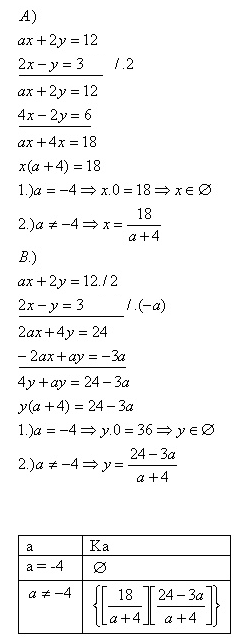sk|cz|

# Parametric Equations

1. Solve and find out the conditions: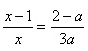Solution: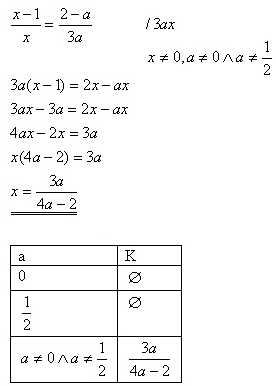2.Solve and find out the conditions: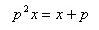Solution: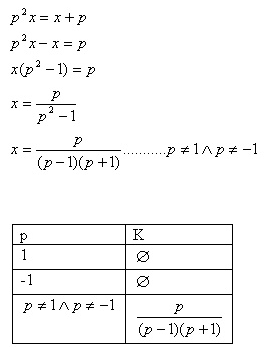3.Solve and find out the conditions: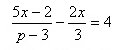Solutions: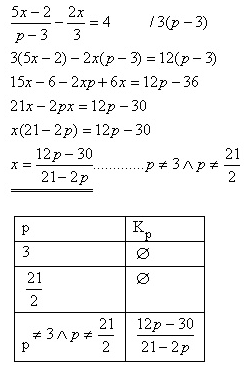4.Solve and find out the conditions: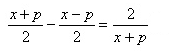Solution: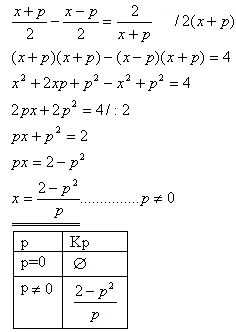5.Solve and find out the conditions: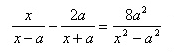Solution: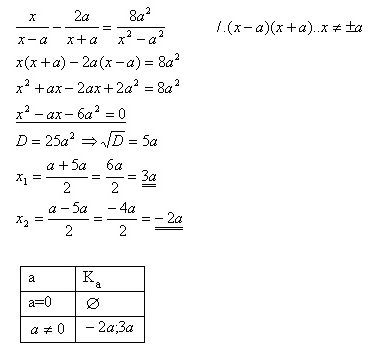6.Solve and find out the conditions: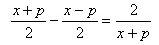Solution: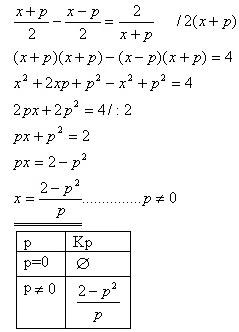7.Solve and discuss the result: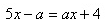Solution: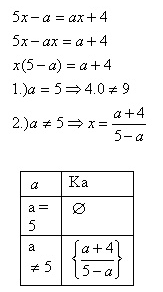8.Solve and discuss the result: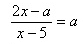Solution: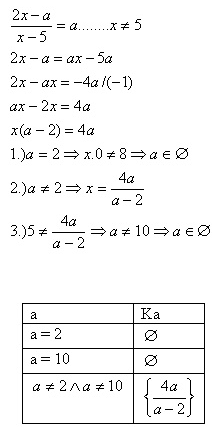9.Solve and discuss the result: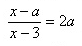Solution: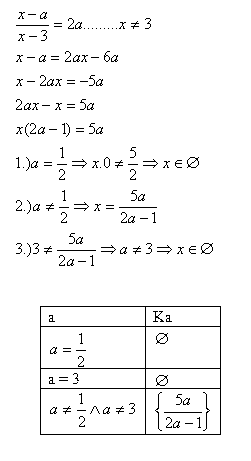10.Solve and discuss the result: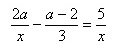Solution: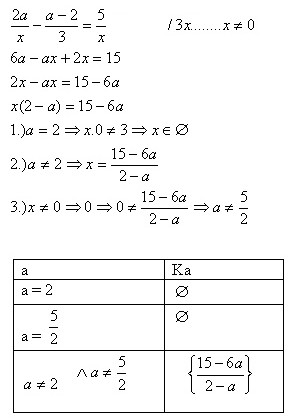11.Solve the set of equations and discuss the result: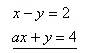Solution: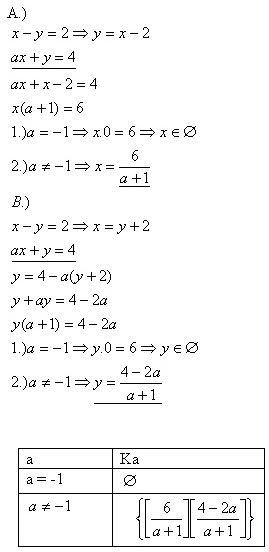12.Solve and discuss the result: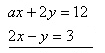Solution: